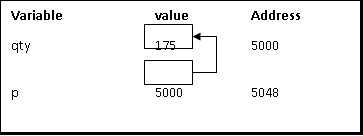# Write a C program demonstrating examples on pointers

A pointer is a variable that stores the address of another variable.

## Features of Pointers

• Pointer saves the memory space.

• The execution time of a pointer is faster because of direct access to memory location.

• With the help of pointers, the memory is accessed efficiently, i.e., memory is allocated and deallocated dynamically.

• Pointers are used with data structures.

## Declaring a pointer

int *p;

It means ‘p’ is a pointer variable that holds the address of another integer variable.

## Initialization of a pointer

Address operator (&) is used to initialize a pointer variable.

For example,

int qty = 175;
int *p;
p= &qty;## Accessing a variable through its pointer

To access the value of the variable, the indirection operator (*) is used.

### Program

Live Demo

#include<stdio.h>
void main(){
//Declaring variables and pointer//
int a=2;
int *p;
//Declaring relation between variable and pointer//
p=&a;
//Printing required example statements//
printf("Size of the integer is %d",sizeof (int));//4//
printf("Value of %d is %d",a,*p);//2//
printf("Value of next address location of %d is %d",a,*(p+1));//Garbage value from (p+1) address//
//Typecasting the pointer//
//Initializing and declaring character data type//
//a=2 = 00000000 00000000 00000000 00000010//
char *p0;
p0=(char*)p;
//Printing required statements//
printf("Size of the character is %d",sizeof(char));//1//
printf("Value of %d is %d",a,*p0);//First byte of value a - 2//
printf("Value of next address location of %d is %d",a,*(p0+1));//Second byte of value a - 0//
}

### Output

Size of the integer is 4
Value of 2 is 2
Value of next address location of 2 is 10818512
Size of the character is 1
Value of 2 is 2
Value of next address location of 2 is 0
Address of next address location of 2 is 6422029

Updated on: 06-Mar-2021

793 Views